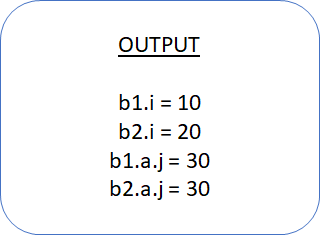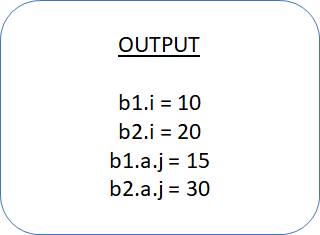## Shallow Copy VS Deep Copy# Shallow Copy VS Deep Copy

As we know that Classes contains Properties and Methods. A Class may also contain other Class Instantiation as a part of it. All these variables inside a Class got initialized once the Class is constructed. How does that happen, lets see using the following example:

``````class A;
int j = 15;
endclass

class B;
int i = 10;
A a = new;
endclass

program main;
B b1, b2;
initial begin
b1 = new;
b2 = new b1; //Shallow copy of object b1 copies first level of properties to b2
b2.i = 20;
b2.a.j = 30; //Assigns 30 to variable “j” shared by both b1 & b2
\$display("b1.i = %0d",b1.i);
\$display("b2.i = %0d",b2.i);
\$display("b1.a.j = %0d",b1.a.j);
\$display("b2.a.j = %0d",b2.a.j);
end
endprogram
``````Here we are having two Classes named “Class A” & “Class B“. Class A instantiates another Class i.e. B. It means, Class A is having two variables inside i.e. integer variable i and Class variable a of type Class “A”. Here we created two variables of Class B i.e. b1 & b2. First one Object is constructed and its Handle is assigned to b1. Another Object is created by doing the “Shallow” copy using “new” construct. In this case, two Object occupy different space in the memory. An important thing to note is that only first level of Properties are copied using the Shallow copy not the Object inside the Parent class. In our example given above, Object of Class type A is constructed automatically when we construct the Object of B Class. During Shallow copy Object of class A is not copied just like integer variable “i” is copied. This behavior can be observed in the given example. If we will not assign any value to “A2.b“, same value i.e 10. Notice we’ve only assigned “b2.a.j” the value 30. But if we access using the Handle “b1.a.j“, we got the same value i.e. 30. It shows that Object a is not copied separately but commonly referenced by both the variables.

Deep Copy:

In Shallow Copy, Objects will not be copied, only their handles will be copied. In order to perform “Deep Copy”, custom method must be added. in the custom method new object is created and all the class properties will be copied to new handle and new handle will be returned.In other words  “Deep Copy: Complete copy of entire class including all contained objects”

Let’s understand better through following example

``````class A;
int j = 15;
endclass

class B;
int i = 10;
A a = new;

function void copy(B obj_b); //In order to do a “deep” copy, a custom copy method must be created
this.i = obj_b.i;
this.a = new obj_b.a;
endfunction : copy
endclass

program main;

B b1=new, b2=new;

initial begin
b2.copy(b1);
b2.i = 20;
b2.a.j = 30;
\$display("b1.i = %0d",b1.i);
\$display("b2.i = %0d",b2.i);
\$display("b1.a.j = %0d",b1.a.j);
\$display("b2.a.j = %0d",b2.a.j);
end
endprogram
``````In the above example you can see that the Class B and Class A we defined, we’ve inserted a copy() function in Class B which copies the Class Properties “i” and “a” as we wanted them to be copied. We must write copy() method for all our Subclass & call its copy() method. Since copy() method is a custom method so it gives better control in terms of choosing what to copy and what Properties to exclude from the copy.In above example we can see that there is a copy of all the Properties including the instantiated Class A as well. As b1.a.i is assigned a value of 30. b2.a.j will show the default value 15 while running the above code. It shows that two different Objects are constructed for Class A yet in Shallow copy only one Object was constructed for Class A.

Latest Posts

Hot items

• 0.00